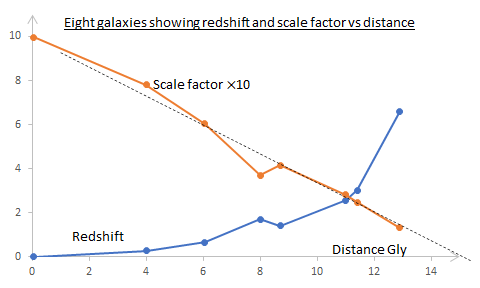## Tuesday, 9 March 2021

### Redshifts and DistancesStill working on the magic Killing tensor $K_{\mu\nu}=a^2\left(g_{\mu\nu}+U_\mu U_\nu\right)$ in FLRW spacetime:
• We use $K_{\mu\nu}$ to show that interstellar gas cools in an expanding universe.
• Then we use it to show that as the universe expands the observed frequency of a photon will decrease.
• Cosmologist measure the redshift, $z$, of distant objects and we show how this tells us the scale factor $a$ when the object emitted the light being measured.
• I then looked up some galactic distances and redshifts to compare them (pictured). Extrapolating the graph indicates the size of the visible universe is ~15 Gly.
• Carroll then seems to ramble a bit and goes back to a non-relativistic redshift. The history of Doppler and Hubble, which he does not mention, is quite interesting.
• Getting back on track Carrol shows that the nearby universe can be thought of as flat and we are able to use proper distance meaningfully and derive Hubble's law. I think he is overcomplicated.
• Next step other distances!

## Tuesday, 2 March 2021

### A Killing Tensor in FLRW spacetime

God (or the universe) was playing tricks on me last week. In section 8.5 we are looking at redshifts and distances. The latter are more complicated than you might thing.

I have got to the end of the first paragraph in this section and ignited a controversy on Physics Forums about $a$ the scale factor which tells you how much the universe is expanding. The question is: Is the scale factor a scalar?

We start in an FLRW universe with metric$${ds}^2=-{dt}^2+a^2\left(t\right)\left[\frac{{\rm dr}^2}{1-\kappa r^2}+r^2{d\theta}^2+r^2\sin^2{\theta}{d\phi}^2\right]$$First Carroll invents a tensor$$K_{\mu\nu}=a^2\left(g_{\mu\nu}+U_\mu U_\nu\right)$$where $U^\mu=\left(1,0,0,0\right)$ is the 4-velocity of (all) comoving observers. Carroll says it satisfies $$\nabla_{(\sigma}K_{\mu\nu)}=0$$"as you can check" and therefore it is a Killing tensor. I accepted the challenge and it took me a few days.

I was not sure if the scale factor $a$ is a scalar. If it's not then it is doubtful whether $K_{\mu\nu}$ is even a tensor. If $a$ is not a scalar but somehow $K_{\mu\nu}$ is a tensor then we must calculate $\nabla_\sigma K_{\mu\nu}$ by expanding the covariant derivative:$$\nabla_\sigma K_{\mu\nu}=\mathrm{\partial}_\sigma K_{\mu\nu}-\Gamma_{\sigma\mu}^\lambda K_{\lambda\nu}-\Gamma_{\sigma\nu}^\lambda K_{\mu\lambda}$$If $a$ is a scalar then we can use the Leibniz rule: $$\nabla_\sigma K_{\mu\nu}=\left(g_{\mu\nu}+U_\mu U_\nu\right)\nabla_\sigma\left(a^2\right)+a^2\nabla_\sigma\left(g_{\mu\nu}+U_\mu U_\nu\right)$$I used Leibniz first and failed. Then I was inspired by a simpler version on physics.stackexchange and got the right answer using the first formula. That made me doubt that $a$ was a scalar. So I asked about it on Physics Forums. Then I found my error in the Leibniz method and it gave the right answer! So now I think that $a$ is a scalar after all. The Leibniz way is a good deal more efficient than the first.  The discussion continues and I think that PeterDonis has had the last word although vanhees71 is still wriggling at ##11. He surrenders at #13.